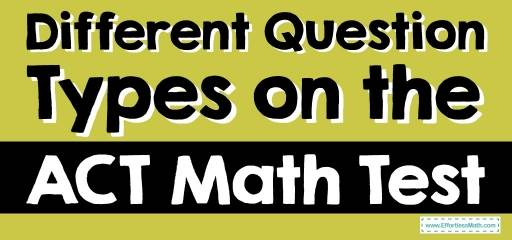# Different Question Types on the ACT Math TestWhen applying for colleges many will take the ACT to submit their applications.

A standardized test that is equal and often interchangeable with the SAT, it is taken at the end of high school to show colleges the ability of each student to complete tasks and questions that apply to their college courses.

Often mandatory in four-year courses, they may not be required for two-year diplomas or degree programs. Often the choice is up to the student whether they take the SAT or the ACT, and either is acceptable and can be up to half of the reason for their acceptance to the college.

Comprised of four sections; math, reading, science, and English, there may also be an additional essay question.

The four main parts of the test are presented in multiple-choice format with a set number of questions and possible answers.

The math portion will have 60 multiple-choice questions and will allow 60 minutes to complete the exam, allowing you one minute for each question.

The Absolute Best Book to Ace the ACT Math Test

## Maths Included

The math portion is compiled of three different math types; algebra, geometry, and trigonometry.

The algebra section has 14 questions based on pre-algebra, 10 created in elementary algebra, and another 9 questions created for intermediate algebra. This mix covers number theory, fractions, decimals, inequalities, ratios and percentages, quadratic equations, square roots, exponents, and more.

The geometry section includes 14 questions around angles, lengths, shapes, volume, and area, and 9 that are based on the slope, distance, parallel lines versus perpendicular lines, and graphing questions. Along with these are 4 trigonometry questions that cover the full topic.

## Calculator Rules

Due to the topics and questions that are included in the exam, these are completed with a scientific calculator, and there is a list provided for which calculators are approved and admitted to the exam so you can plan. Know your calculator well for the exam, and practice with it so you can easily complete many calculations.

It is also necessary to ensure that you have memorized all of the top formulas as they will not be provided in the exam and the memory for each calculator must be emptied before the exam. Memorizing the formulas and practicing how to enter them into the calculator you will be taking with you to the exam is a great way to prepare.

Best ACT Math Prep Resource

## Practice Tests

Completing the online practice tests and available study guides will prepare you best for the test. These often use questions that have been included on the exam in the past for an accurate portrayal of the exam.

They include the same wording that will be used in the exam so you will be prepared to understand what the question is asking and how to work out the solution.

The ACT exam can be key in getting into your first-choice college, and preparing for it with the correct calculator, formulas, and practice exams is the best way to ensure success.

The Best Books to Ace the ACT Math Test

## More from Effortless Math for ACT Test …

### Are you looking for a FREE ACT Math course to help you prepare for your test?

Check out our Ultimate ACT Math Course.

Have a look at our comprehensive ACT Math Worksheets to help you practice and prepare for the ACT Math test.

### Want to review the most common ACT Math formula?

Here is our complete list of ACT Math formulas.

### Looking for FREE ACT Math websites to find free online resources?

Here is our complete list of the Top 10 Free Websites for ACT Math Preparation.

Have a look at our Full-Length ACT Math Practice Test and Free ACT Math Practice Test.

### Do you know the key differences between SAT Math and ACT Math?

Find your answer here: SAT Math vs. ACT Math: the key differences.

The Perfect Prep Books for the ACT Math Test

## Have any questions about the ACT Test?

### What people say about "Different Question Types on the ACT Math Test - Effortless Math: We Help Students Learn to LOVE Mathematics"?

No one replied yet.

X
52% OFF

Limited time only!

Save Over 52%

SAVE \$40

It was \$76.99 now it is \$36.99